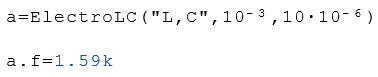# Class ElectroLC

Calculation of circuits with capacitors and coils

## Description

The class $$ElectroLC$$ contains methods for the calculating circuits with capacitors and coils. This class is an extension of the class Electro. This means that all properties and methods of the class Electro are also included in this class.

## Eigenschaften

#### Description

U

Voltage in Volt

I

Current in amperes

P

Power in Watts

R

Resistance in ohms

G

Conductance in Siemens

Q

Charge in Coulomb

t

Time in seconds - time to calculate $$Ut$$ oder $$It$$

f

Frequency in Hertz

ω

omega

Angular frequency

λ

lamda

Wavelength in meters

Τ

Tau

Time constant in seconds

L

Inductance in Henry

C

W

Energy of a capacitor in joules

Z

Impedance in ohms

Ut

Voltage at a specific time

It

Current at any given time

φ

phi

Phase angle in radian## Examples

Example 1Example 2Example 3